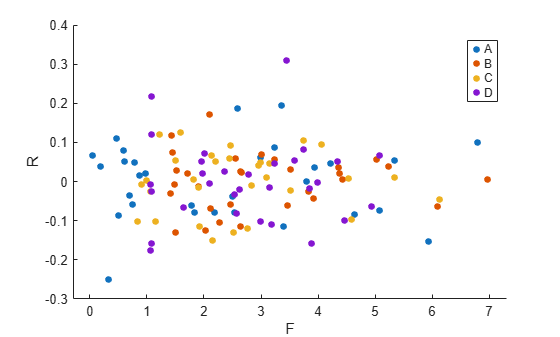# residuals

Residuals of fitted linear mixed-effects model

## Syntax

``R = residuals(lme)``
``R = residuals(lme,Name,Value)``

## Description

example

````R = residuals(lme)` returns the raw conditional residuals from a fitted linear mixed-effects model `lme`.```

example

````R = residuals(lme,Name,Value)` returns the residuals from the linear mixed-effects model `lme` with additional options specified by one or more `Name,Value` pair arguments.For example, you can specify Pearson or standardized residuals, or residuals with contributions from only fixed effects.```

## Examples

collapse all

`load('weight.mat');`

`weight` contains data from a longitudinal study, where 20 subjects are randomly assigned to 4 exercise programs, and their weight loss is recorded over six 2-week time periods. This is simulated data.

Store the data in a table. Define `Subject` and `Program` as categorical variables.

```tbl = table(InitialWeight,Program,Subject,Week,y); tbl.Subject = nominal(tbl.Subject); tbl.Program = nominal(tbl.Program);```

Fit a linear mixed-effects model where the initial weight, type of program, week, and the interaction between the week and type of program are the fixed effects. The intercept and week vary by subject.

`lme = fitlme(tbl,'y ~ InitialWeight + Program*Week + (Week|Subject)');`

Compute the fitted values and raw residuals.

```F = fitted(lme); R = residuals(lme);```

Plot the residuals versus the fitted values.

```plot(F,R,'bx') xlabel('Fitted Values') ylabel('Residuals')```Now, plot the residuals versus the fitted values, grouped by program.

```figure(); gscatter(F,R,Program)```The residuals seem to behave similarly across levels of the program as expected.

`load carbig`

Store the variables for miles per gallon (MPG), acceleration, horsepower, cylinders, and model year in a table.

`tbl = table(MPG,Acceleration,Horsepower,Cylinders,Model_Year);`

Fit a linear mixed-effects model for miles per gallon (MPG), with fixed effects for acceleration, horsepower, and the cylinders, and potentially correlated random effects for intercept and acceleration grouped by model year.

`lme = fitlme(tbl,'MPG ~ Acceleration + Horsepower + Cylinders + (Acceleration|Model_Year)');`

Compute the conditional Pearson residuals and display the first five residuals.

```PR = residuals(lme,'ResidualType','Pearson'); PR(1:5)```
```ans = 5×1 -0.0533 0.0652 0.3655 -0.0106 -0.3340 ```

Compute the marginal Pearson residuals and display the first five residuals.

```PRM = residuals(lme,'ResidualType','Pearson','Conditional',false); PRM(1:5)```
```ans = 5×1 -0.1250 0.0130 0.3242 -0.0861 -0.3006 ```

`load carbig`

Store the variables for miles per gallon (MPG), acceleration, horsepower, cylinders, and model year in a table.

`tbl = table(MPG,Acceleration,Horsepower,Cylinders,Model_Year);`

Fit a linear mixed-effects model for miles per gallon (MPG), with fixed effects for acceleration, horsepower, and the cylinders, and potentially correlated random effects for intercept and acceleration grouped by model year.

`lme = fitlme(tbl,'MPG ~ Acceleration + Horsepower + Cylinders + (Acceleration|Model_Year)');`

Draw a histogram of the raw residuals with a normal fit.

```r = residuals(lme); histfit(r)```Normal distribution seems to be a good fit for the residuals.

Compute the conditional Pearson and standardized residuals and create box plots of all three types of residuals.

```pr = residuals(lme,'ResidualType','Pearson'); st = residuals(lme,'ResidualType','Standardized'); X = [r pr st]; boxplot(X)```Red plus signs show the observations with residuals above or below $q3+1.5\left(q3-q1\right)$ and $q1-1.5\left(q3-q1\right)$, where $q1$ and $q3$ are the 25th and 75th percentiles, respectively.

Find the observations with residuals that are 2.5 standard deviations above and below the mean.

`find(r > mean(r,'omitnan') + 2.5*std(r,'omitnan'))`
```ans = 7×1 62 252 255 330 337 341 396 ```
`find(r < mean(r,'omitnan') - 2.5*std(r,'omitnan'))`
```ans = 3×1 119 324 375 ```

## Input Arguments

collapse all

Linear mixed-effects model, specified as a `LinearMixedModel` object constructed using `fitlme` or `fitlmematrix`.

### Name-Value Arguments

Specify optional pairs of arguments as `Name1=Value1,...,NameN=ValueN`, where `Name` is the argument name and `Value` is the corresponding value. Name-value arguments must appear after other arguments, but the order of the pairs does not matter.

Before R2021a, use commas to separate each name and value, and enclose `Name` in quotes.

Example: ```R = residuals(lme,'ResidualType','Pearson');```

Indicator for conditional residuals, specified as the comma-separated pair consisting of `'Conditional'` and one of the following.

 `True` Contribution from both fixed effects and random effects (conditional) `False` Contribution from only fixed effects (marginal)

Example: `'Conditional,'False'`

Residual type, specified by the comma-separated pair consisting of `ResidualType` and one of the following.

Residual TypeConditionalMarginal
`'Raw'`

`${r}_{i}^{C}={\left[y-X\stackrel{^}{\beta }-Z\stackrel{^}{b}\right]}_{i}$`

`${r}_{i}^{M}={\left[y-X\stackrel{^}{\beta }\right]}_{i}$`

`'Pearson'`

`$p{r}_{i}^{C}=\frac{{r}_{i}^{C}}{{\sqrt{\left[{\stackrel{^}{Var}}_{y,b}\left(y-X\beta -Zb\right)\right]}}_{ii}}$`

`$p{r}_{i}^{M}=\frac{{r}_{i}^{M}}{\sqrt{{\left[{\stackrel{^}{Var}}_{y}\left(y-X\beta \right)\right]}_{ii}}}$`

`'Standardized'`

`$s{t}_{i}^{C}=\frac{{r}_{i}^{C}}{\sqrt{{\left[{\stackrel{^}{Var}}_{y}\left({r}^{C}\right)\right]}_{ii}}}$`

`$s{t}_{i}^{M}=\frac{{r}_{i}^{M}}{\sqrt{{\left[{\stackrel{^}{Var}}_{y}\left({r}^{M}\right)\right]}_{ii}}}$`

For more information on the conditional and marginal residuals and residual variances, see `Definitions` at the end of this page.

Example: `'ResidualType','Standardized'`

## Output Arguments

collapse all

Residuals of the fitted linear mixed-effects model `lme`returned as an n-by-1 vector, where n is the number of observations.

collapse all

### Conditional and Marginal Residuals

Conditional residuals include contributions from both fixed and random effects, whereas marginal residuals include contribution from only fixed effects.

Suppose the linear mixed-effects model `lme`has an n-by-p fixed-effects design matrix X and an n-by-q random-effects design matrix Z. Also, suppose the p-by-1 estimated fixed-effects vector is $\stackrel{^}{\beta }$, and the q-by-1 estimated best linear unbiased predictor (BLUP) vector of random effects is $\stackrel{^}{b}$. The fitted conditional response is

`${\stackrel{^}{y}}_{Cond}=X\stackrel{^}{\beta }+Z\stackrel{^}{b},$`

and the fitted marginal response is

`${\stackrel{^}{y}}_{Mar}=X\stackrel{^}{\beta },$`

`residuals` can return three types of residuals: raw, Pearson, and standardized. For any type, you can compute the conditional or the marginal residuals. For example, the conditional raw residual is

`${r}_{Cond}=y-X\stackrel{^}{\beta }-Z\stackrel{^}{b},$`

and the marginal raw residual is

`${r}_{Mar}=y-X\stackrel{^}{\beta }.$`

For more information on other types of residuals, see the `ResidualType` name-value pair argument.

## Version History

Introduced in R2013b